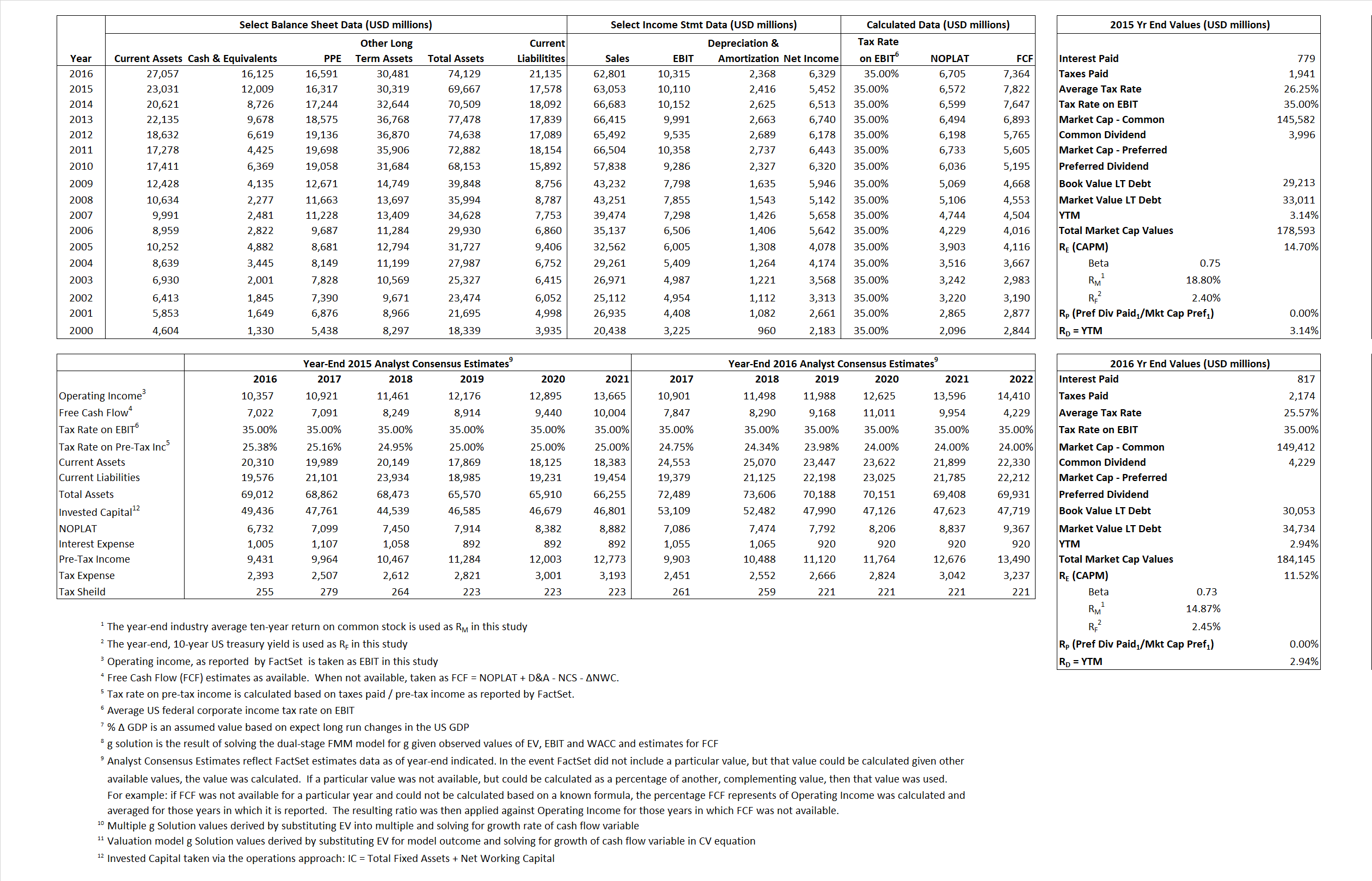# Pepsi Co

## Analyst Listing

The following analysts provide coverage for the subject firm as of May 2016:

 Broker Analyst Analyst Email Bernstein Research Ali Dibadj ali.dibadj@bernstein.com BMO Capital Markets Amit Sharma amit.sharma@bmo.com CRT Capital Group April Scee ascee@sterneageerct.com Deutsche Bank Research Bill Schmitz Jr. william.schmitz@db.com Wells Fargo Securities Bonnie Herzog bonnie.herzog@wellsfargo.com Consumer Edge Research Brett Cooper bcooper@consumeredgeresearch.com Athlos Research Jonathan P. Feeney jonathan@athlosresearch.com First Global Stockbroking Kavita Thomas kavita.thomas@fglobal.com Jefferies Kevin Grundy kgrundy@jefferies.com Stifel Nicolaus Mark D. Swartzberg mswartzberg@stifel.com RBC Capital Markets Nik Modi nik.modi@rbccm.com Susquehanna Financial Group Pablo Zuanic pablo.zuanic@sig.com Evercore ISI Robert Ottenstein robert.ottenstein@evercoreisi.com Cowen & Company Vivien Azer vivien.azer@cowen.com

## Primary Input Data## Derived Input Data

### Equational Form

Net Operating Profit Less Adjusted Taxes NOPLAT 6,572 6,705$NOPLAT\, =\, EBIT\, x\, (1 \,-\, Avg \,\,Tax\,\, Rate\,\, on\,\, EBIT)$
Free Cash Flow FCF 7,822 7,364$FCF\,=NOPLAT\,+\,Non-Cash\,Expenses-\Delta NWC\,-\,NCS$
Tax Shield TS 205 209$TS\,=\,Interest\,\,Paid\,\,x\,\, Avg \,\,Tax\,\,Rate\,\, on\,\, Pre-Tax\,\, Income$
Invested Capital IC  52,089 52,994$IC\,=\,Fixed\,\,Operating\,\,Assets\,\,+\,\,Net\,\, Working\,\, Capital$
Return on Invested Capital ROIC  12.62% 12.65%$ROIC\,=\,\frac { NOPLAT }{ IC }$
Net Investment NetInv 2,088 3,273$NetInv\,=\,{ {IC}_{1}}-{{IC}_{0}}+Depreciation$
Investment Rate IR  31.77% 48.82%$IR\,=\,\frac {NetInv}{NOPLAT}$
Weighted Average Cost of Capital
WACCMarket 12.41%  9.76%$WACC\,=\,\frac { E }{ V } { R }_{ E }\,+\,\frac { P }{ V } { R }_{ P }\,+\,\frac { D }{ V } { R }_{ D }\left( 1- Avg\,\, Tax\,\,Rate\,\,on\,\,Pre-Tax\,\,Income \right)$
WACCBook   6.55%   6.23%
Enterprise value
EVMarket 166,584  168,020$EV\,=\,Market\,\,Cap\,\,Equity\,+\,\,Long\,\,Term\,\,Debt\,-\,Cash$
EVBook  158,670  163,340
Long-Run Growth
g = IR x ROIC
4.01%    6.18% Long-run growth rates of the income variable are used in the Continuing Value portion of the valuation models.
g = %$\Delta$ GDP    2.50%    2.50%
Margin from Operations M   16.03%   16.42%$M\,\,=\,\,\frac{EBIT}{SALES}$
Depreciation/Amortization Rate D  19.29%   18.67%$D\,\,=\,\,\frac{D+A}{EBITDA}$

## Valuation Multiple Outcomes

The outcomes presented in this study are the result of original input data, derived data, and synthesized inputs.

### model g solution

12/31/2015 12/31/2016 12/31/2015 12/31/2016 12/31/2015 12/31/2016

EV/SALES$\frac {EV}{Sales} \,= \,\frac{ROIC\, -\, g}{ROIC\,(WACC\,-\,g)}\,(1\,-\,T)\,(M)$

2.64 2.68  12.32%  8.42% 12.36%  9.04%

EV/EBITDA$\frac {EV}{EBITDA} \,= \,\frac{ROIC\, -\, g}{ROIC\,(WACC\,-\,g)}\,(1\,-\,T)\,(1\,-\,D)$

13.30 13.25 12.32% 8.42% 12.36% 9.04%

EV/NOPLAT$\frac {EV}{NOPLAT} \,= \,\frac{ROIC\, -\, g}{ROIC\,(WACC\,-\,g)}$

25.35 25.06 12.32% 8.42% 12.36% 9.04%

EV/FCFOPS$\frac {EV}{FCF_{OPS}} \,= \,\frac{ROIC\, -\, g}{ROIC\,(WACC\,-\,g)}\,(1\,-\,T)$

21.30 22.82 12.32% 8.42% 12.36% 9.04%

EV/EBIT$\frac {EV}{EBIT} \,= \,\frac{ROIC\, -\, g}{ROIC\,(WACC\,-\,g)}\,(1\,-\,T)$

16.48 16.29 12.32% 8.42% 12.36% 9.04%

EV/IC$\frac {EV}{IC} \,= \,\frac{ROIC\, -\, g}{WACC\,-\,g}$

3.20 3.17 12.32% 8.42% 12.36% 9.04%